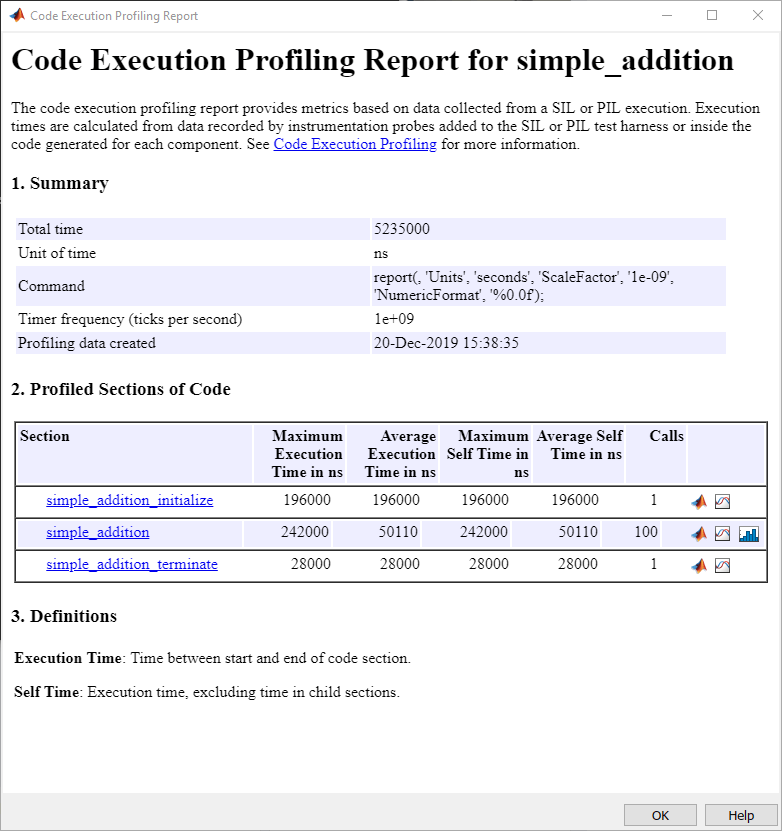# Processor-in-the-Loop Verification of MATLAB Functions

This example shows how to use Embedded Coder® Support Package for ARM Cortex-A® Processors for processor-in-the-loop (PIL) verification of MATLAB® functions on an ARM Cortex-A9 (QEMU) emulator.

### Introduction

This example shows how to generate a PIL MEX function from a MATLAB function. When you run the PIL MEX function, the generated C-code from your MATLAB function runs on the ARM Cortex-A9 (QEMU) emulator, transferring the results back to MATLAB for numerical verification. Using this process, you can profile the code execution. For more information on PIL, see Code Verification Through Software-in-the-Loop and Processor-in-the-Loop Execution. For more information on the QEMU emulator, see the QEMU website.

### Create and Run PIL MEX Function

This section shows how to create a PIL MEX equivalent of a sample function to be run on the emulated hardware. Create and navigate into a new folder on a local drive. This folder stores the sample function and generated PIL code. Create a function file, `simple_addition.m`, containing a function that adds two inputs and returns the result. The `%#codegen` directive in the function indicates that the MATLAB code is intended for code generation.

```function y = simple_addition(u1,u2) %#codegen % Copyright 2015 The MathWorks, Inc. y = u1 + u2; end ```

Create a coder configuration object by using the `coder.config` function. Set the properties of the cofiguration object as shown.

```cfg = coder.config('lib','ecoder',true); cfg.VerificationMode = 'PIL'; cfg.CodeExecutionProfiling = 1; cfg.BuildConfiguration = 'Faster Runs'; ```

Create a QEMU hardware object by using the `coder.hardware` function, and then attach that object to the coder configuration object. The hardware object signals the `codegen` function to generate a PIL executable that runs on ARM Cortex-A9 (QEMU) emulator and a PIL MEX function to run the executable on the emulator.

```cfg.Hardware = coder.hardware('ARM Cortex-A9 (QEMU)'); ```

Use the `codegen` function with these specified input arguments for the `simple_additional` function to generate a PIL MEX function named `simple_addition_pil` in the current folder.

```codegen('-config ',cfg,... 'simple_addition','-args',{single(zeros(1024,1)),single(zeros(1024,1))},... '-report'); ```

Run the PIL MEX function to compare its behavior to that of the original MATLAB function and to check for run-time errors.

```u1 = single(rand(1024,1)); u2 = single(rand(1024,1)); y_expected = simple_addition(u1,u2); y_PIL = simple_addition_pil(u1,u2); ```

To verify the numerical accuracy of the generated code, use the `assert` function to check that the MATLAB results are equivalent to those of the PIL MEX function.

```assert(norm(y_expected - y_PIL) == 0); ```

### Profile Generated Code

To enable code execution profiling of a function, set the `CodeExecutionProfiling` property of the coder configuration object to `true`. Profiling instruments the generated code with timing information. When the PIL MEX function is cleared from memory, the profiling results get transferred to MATLAB. To accumulate profiling results, run the `simple_addition` function 100 times in a loop.

```for k=1:100 y = simple_addition_pil(u1,u2) end clear simple_addition_pil ```

To open the profiling report, use the `getCoderExecutionProfile` and `report` functions.

```report(getCoderExecutionProfile('simple_addition')) ```### Use Code Replacement Library (CRL)

To take advantage of an optimized CRL for ARM Cortex-A processors, you can rebuild the PIL MEX function with the ARM Cortex-A CRL.

```cfg = coder.config('lib','ecoder',true); cfg.VerificationMode = 'PIL'; cfg.CodeExecutionProfiling = 1; cfg.BuildConfiguration = 'Faster Runs'; cfg.CodeReplacementLibrary = 'ARM Cortex-A'; % Use CRL ```

Run the `simple_addition` function 100 times in a loop to accumulate profiling results. Then, open the profiling report.

```for k=1:100 y = simple_addition_pil(u1,u2) end clear simple_addition_pil report(getCoderExecutionProfile('simple_addition')) ```

Compare the profiling results to those obtained from the previous section.